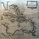324 vizualizações
This is similar to my earlier GBPNZD pairs slope indicator. This indicator determines if the close of a pair (highly correlated pairs to GBPNZD ) is higher or lower than a common displaced moving average. If it is above, a count of 1 * corr*corr factor is assinged. For you programmers I multiply by corr*corr to erase the negative corr factor as the IF THEN statement will assign a 1 or -1. Note: I could not find an absolute value function, so corr^2 clears it. The spirit of this indicator, as with my others, is to sniff out underling movement in GBP or NZD to predict a trend change. The idea of the count comes from counting cards in blackjack. Toy around with chart time frames and the inputs to get a feel for it.

```//@version=2
study(title="GBPNZD hilo count avet",
shorttitle="GBPNZD hilo count ave")
l = input(title="Length", type=integer,defval=30, minval=5)
d = input(title = "displacement", type=integer, defval=30, minval=1)
cl = input(title="Length", type=integer,defval=30, minval=5)
smoother = input(title="EMA length", type=integer,defval=5, minval=5)
p0 = input(title="Other data series", type=symbol,defval="FX_IDC:gbpnzd")
p1 = input(title="Other data series", type=symbol,defval="FX_IDC:gbpaud")
p2 = input(title="Other data series", type=symbol,defval="FX_IDC:gbpcad")
p3 = input(title="Other data series", type=symbol,defval="FX_IDC:gbpchf")
p4 = input(title="Other data series", type=symbol,defval="FX_IDC:gbpjpy")
p5 = input(title="Other data series", type=symbol,defval="FX_IDC:gbpusd")
p6 = input(title="Other data series", type=symbol,defval="FX_IDC:nzdusd")
p7 = input(title="Other data series", type=symbol,defval="FX_IDC:eurjpy")
p8 = input(title="Other data series", type=symbol,defval="FX_IDC:eurnzd")
p9 = input(title="Other data series", type=symbol,defval="FX_IDC:eurgbp")
p10 = input(title="Other data series", type=symbol,defval="FX_IDC:nzdchf")
p11 = input(title="Other data series", type=symbol,defval="FX_IDC:nzdcad")
// Get the additional data series
s0= security(p0, period, close)
s1= security(p1, period, close)
s2= security(p2, period, close)
s3= security(p3, period, close)
s4= security(p4, period, close)
s5= security(p5, period, close)
s6= security(p6, period, close)
s7= security(p7, period, close)
s8= security(p8, period, close)
s9= security(p9, period, close)
s10= security(p10, period, close)
s11= security(p11, period, close)
// Calculate correlation and slopes
corr0 = correlation(close, s0, l)
corr1 = correlation(close, s1, l)
corr2 = correlation(close, s2, l)
corr3 = correlation(close, s3, l)
corr4 = correlation(close, s4, l)
corr5 = correlation(close, s5, l)
corr6 = correlation(close, s6, l)
corr7 = correlation(close, s7, l)
corr8 = correlation(close, s8, l)
corr9 = correlation(close, s9, l)
corr10 = correlation(close, s10, l)
corr11 = correlation(close, s11, l)
sma0 = sma(s0, l)[d]
sma1 = sma(s1, l)[d]
sma2 = sma(s2, l)[d]
sma3 = sma(s3, l)[d]
sma4 = sma(s4, l)[d]
sma5 = sma(s5, l)[d]
sma6 = sma(s6, l)[d]
sma7 = sma(s7, l)[d]
sma8 = sma(s8, l)[d]
sma9 = sma(s9, l)[d]
sma10 = sma(s10, l)[d]
sma11 = sma(s11, l)[d]
m0 = iff(s0>sma0,1,-1)*corr0*corr0
m1 = iff(s1>sma1,1,-1)*corr1*corr1
m2 = iff(s2>sma2,1,-1)*corr2*corr2
m3 = iff(s3>sma3,1,-1)*corr3*corr3
m4 = iff(s4>sma4,1,-1)*corr4*corr4
m5 = iff(s5>sma5,1,-1)*corr5*corr5
m6 = iff(s6>sma6,1,-1)*corr6*corr6
m7 = iff(s7>sma7,1,-1)*corr7*corr7
m8 = iff(s8>sma8,1,-1)*corr8*corr8
m9 = iff(s9>sma9,1,-1)*corr9*corr9
m10 = iff(s10>sma10,1,-1)*corr10*corr10
m11 = iff(s11>sma11,1,-1)*corr11*corr11
c = m0+m1+m2+m3+m4+m5+m6+m7+m8+m9+m10+m11
// m0+m1+m2+m3+m4+m5+m6+m7+m8+m9+m10+m11
csmooth = sma(c, smoother)
cave = sma(c, cl)
// Plot values
//plot(series=c,title="Count")
plot(series=csmooth, color=blue, linewidth=1, title="Count")
plot(series=cave, color=orange, linewidth=3 ,title="Count Average")
hline(0, color=red)```

## ComentáriosError in 59-70, should only have one *corr# not *corr#*corr#
Resposta